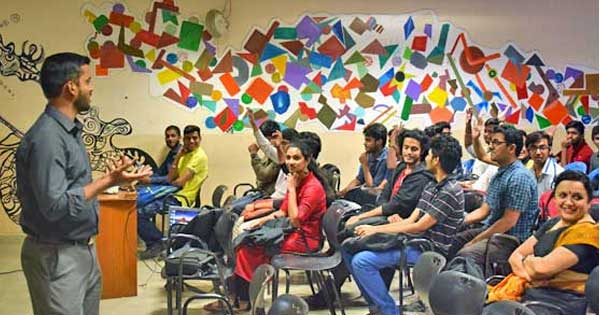## JAIN (Deemed-to-be University) News#### A Session on Mathematical Biology by Alumnus Ujwal P RajIn the Inspiring Alumni session organized by the Department of Mathematics, School of Sciences, Jain (Deemed-to-be University), Ujwal P Raj spoke on the topic Mathematical Biology. The alumnus discussed the basic mathematics required at the college level and the concepts of various theorems and formulae. He briefed on the mathematical background highlighting the reason behind the hexagonal shape of the honey comb.

The speaker focused on the application of mathematics such as predator-prey models which are arguably the building blocks of the bio and ecosystems as biomasses are grown out of their resource masses and this is co-related functionally with mathematics. He explained another application of mathematics which is the Euler method, the most basic explicit method for numerical integration of ordinary differential equations and also the simplest Runge–Kutta method.

Another example related to the application of mathematics discussed by the speaker is Dijkstra’s algorithm that is basically an algorithm for finding the shortest paths between nodes in a graph, which may represent, for example, road networks. It was conceived by computer scientist Edsger W. Dijkstra in 1956 and was published three years later.

The informative session concluded with a vote of thanks followed by the felicitation to the alumnus.